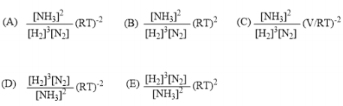# Problem: Consider the Haber process, 3 H2(g) + N2(g) ↔ 2 NH3(g). What is the correct expression for Kp of those below? (all concentrations are at equilibrium)a. Answer Ab. Answer Bc. Answer Cd. Answer De. Answer E

###### FREE Expert Solution

We are being asked to identify Kp expression using the concentrations.

3 H2(g) + N2(g) ↔ 2 NH3(g)

When dealing with equilibrium:

Kc → equilibrium units are in molarity
Kp → equilibrium units in terms of pressure

Kp and Kc are related to one another by the following equation below:

$\overline{){{\mathbf{K}}}_{{\mathbf{p}}}{\mathbf{=}}{{\mathbf{K}}}_{{\mathbf{c}}}{\left(\mathbf{RT}\right)}^{\mathbf{∆}\mathbf{n}}}$

87% (359 ratings)###### Problem Details

Consider the Haber process, 3 H2(g) + N2(g) ↔ 2 NH3(g). What is the correct expression for Kp of those below? (all concentrations are at equilibrium)